# Conservation of energy facts for kids

Kids Encyclopedia Facts
This article refers to the law of conservation of energy in physics. For energy resources sustainably, see: Energy conservation.

In physics, the conservation of energy is that energy can not be created or destroyed, it can only be changed from one form to another, such as when electrical energy is changed into heat energy. Formally, it says that the total amount of energy in an isolated system remains constant, although it may change forms, e.g. friction turns kinetic energy into thermal energy. In thermodynamics, the first law of thermodynamics is a statement of the conservation of energy for thermodynamic systems.

From a mathematical point of view, the energy conservation law is a consequence of the shift symmetry of time; energy conservation is a result of the empirical fact that the laws of physics do not change with time itself. Philosophically, this can be stated as "nothing depends on time per se (time itself)".

## Historical information

Ancient philosophers as far back as Thales of Miletus had the idea that there is some underlying substance of which everything is made. But that is not the same as our concept of "mass-energy" today (for example, Thales thought the underlying substance was water). In 1638, Galileo published his analysis of several situations. This included the famous "interrupted pendulum". This can be described (in modernized language) as conservatively converting potential energy to kinetic energy and back again. However, Galileo did not explain the process in modern terms and had not understood the modern concept either. The German Gottfried Wilhelm Leibniz during 1676-1689 attempted a mathematical formulation of the kind of energy which is connected with motion (kinetic energy). Leibniz noticed that in many mechanical systems (of several masses, mi each with velocity vi ),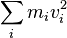$\sum_{i} m_i v_i^2$

was conserved so long as the masses did not interact. He called this quantity the vis viva or living force of the system. The principle represents an accurate statement of the approximate conservation of kinetic energy in situations where there is no friction.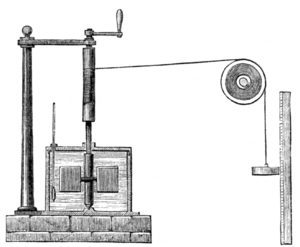Joule's apparatus for measuring the mechanical equivalent of heat. A descending weight attached to a string causes a paddle in water to rotate

Meanwhile, in 1843 James Prescott Joule independently discovered the mechanical equivalent in a series of experiments. In the most famous, now called the "Joule apparatus", a descending weight attached to a string caused a paddle immersed in water to rotate. He showed that the gravitational potential energy lost by the weight in descending was approximately equal to the thermal energy (heat) gained by the water by friction with the paddle.

Over the period 1840-1843, similar work was carried out by engineer Ludwig A. Colding though it was little-known outside his native Denmark.

## Proof

It is easy to see that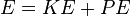$E=KE+PE$

which is also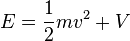$E=\frac{1}{2}mv^2 + V$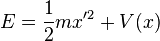$E=\frac{1}{2}mx'^2+V(x)$

Assuming that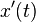$x'(t)$ and that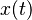$x(t)$, then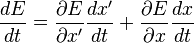$\frac{dE}{dt}=\frac{\partial E}{\partial x'}\frac{dx'}{dt}+\frac{\partial E}{\partial x}\frac{dx}{dt}$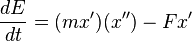$\frac{dE}{dt}=(mx')(x'')-Fx'$

(Since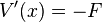$V'(x)=-F$)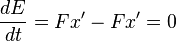$\frac{dE}{dt}=Fx'-Fx'=0$

Therefore, energy does not vary with time.

## Related pages

### Modern accounts

• Goldstein, Martin, and Inge F., 1993. The Refrigerator and the Universe. Harvard Univ. Press. A gentle introduction.

• Stenger, Victor J. (2000). Timeless Reality. Prometheus Books. Especially chpt. 12. Nontechnical.

### Classic accounts

, Chapter 8, "Energy and Thermo-dynamics"

## Images for kidsConservation of energy Facts for Kids. Kiddle Encyclopedia.